Next: The Efficient Test Theorem Up: Pseudo-random number generators Previous: The Quadratic Generator   Contents

## The Next Bit Theorem

It would certainly be undesirable if there were an efficient algorithm which took as input the firstbits of the sequence from the generator and guessed the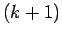-st bit with probability much greater than. We say a generator satisfies the Next Bit Condition if there is no such algorithm.

Theorem 29   If (QRA) is true, the quadratic generator satisfies the Next Bit Condition.

Proof: We will show that an algorithm that could predict the-st bit could be used to distinguish squares from pseudo-squares mod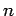.

Let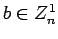. The sequence of lengthcan be considered as coming from the quadratic generator with seed the first term of the sequence. If we take this sequence mod 2 and give it to our predictor, we would get a guess as to whetherwhich has probability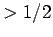of being right. The principle square root of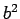is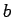ifis a square,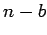ifis a pseudo-square. Since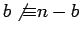mod 2, the information from the predictor gives us a guess as to whetheris a square.Next: The Efficient Test Theorem Up: Pseudo-random number generators Previous: The Quadratic Generator   Contents
Translated from LaTeX by Scott Sutherland
2002-12-14